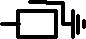# Physics- Spring-Mass-Damper Mechanical Circuits

The spring,mass, damper model allows mechanical systems to be solved in is similar way to electrical circuits using capacitors resistors and inductors.

 Spring Damper MassDifferential equation - Laplace transform - F = k * x F(s) = k * X(s) where k = spring ratio in N/m F = c * dx/dt F(s) = c * s * X(s) where c = damping coefficient in N s /m F = m * d2x/dt2 F(s) = m * s * s * X(s) where m = mass in kg=N*s*s/m

### Example 1equation of motion: m * d2 x/dt2 + k * x =0

solution x = a * cos(wt + alpha)

where w = sqrt(k / m)

a and alpha are constants of integration

###equation of motion: m * d2x/dt2 + c *dx/DT + k * x = 0

### Standard Components

I think that some components, such as motor, gearbox, rack & pinion, etc. should be available to users as standard building blocks, the internal operation of these would not need to be simulated, only the internal behavior.

## Further Information

Example: in car racing game

 metadata block see also: Correspondence about this page Book Shop - Further reading. Where I can, I have put links to Amazon for books that are relevant to the subject, click on the appropriate country flag to get more details of the book or to buy it from them. Commercial Software Shop Where I can, I have put links to Amazon for commercial software, not directly related to the software project, but related to the subject being discussed, click on the appropriate country flag to get more details of the software or to buy it from them.Mathmatica

This site may have errors. Don't use for critical systems.